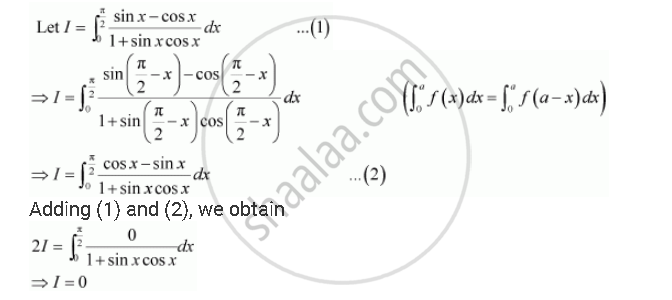Share
Notifications

View all notifications
Books Shortlist
Your shortlist is empty

# Solution for By Using the Properties of Definite Integrals, Evaluate the Integrals Int_0^(Pi/2) (Sin X - Cos X)/(1+Sinx Cos X) Dx - CBSE (Science) Class 12 - Mathematics

Login
Create free account

Forgot password?
ConceptProperties of Definite Integrals

#### Question

By using the properties of definite integrals, evaluate the integrals

int_0^(pi/2) (sin x - cos x)/(1+sinx cos x) dx

#### SolutionIs there an error in this question or solution?

#### Video TutorialsVIEW ALL 

Solution By Using the Properties of Definite Integrals, Evaluate the Integrals Int_0^(Pi/2) (Sin X - Cos X)/(1+Sinx Cos X) Dx Concept: Properties of Definite Integrals.
S